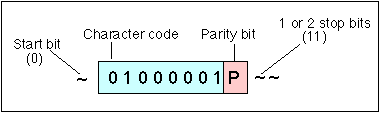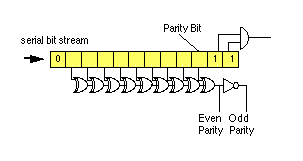# Character Parity

Parity is an error detection technique which is typically used on asynchronous links. It is used to verify the integrity of individual characters (or bytes) within the transmitted stream. When used, each character is protected by a single parity bit which is the logical exclusive or of all the bits in the character. Two types of parity are used: even parity where a 1-parity-bit is sent if there are an even number of 1-bits and odd which is the opposite (i.e. a 1-parity-bit is sent when there are an odd number of 1-bits).Parity bit added to a transmitted character at the transmitter and checked at the receiver

At the receiver the parity is recalculated using either an exclusive-or adder or a Finite State Machine (FSM). An implementation using XOR gates is shown below.Example

Parity may also be calculated in software using a shift register to count the number of '1' bits in each byte. When the calculated parity from the received character does not match the value of the received parity bit, then a parity error is said to have occurred, and the character is normally discarded. This parity check detects any number of odd errors but passes any number of even errors without detecting an error.

Here are some examples to check your understanding. A message "Hello" was sent to a serial command using the C programming statement "printf("Hello\n").

The original sequence of characters are shown in column 1.

The binary bit patterns which are sent are shown in the second column (use an ASCII table to see if this in computer bit order or transmission bit order!)

The third column shows the sequence of received bits, including some transmission errors (can you identify which bits were corrupted?).

The received ASCII characters and the parity condition are shown in the final columns. (Can you validate that the parity logic detects these errors?)

```H S010010011SS S010010011SS H Valid 'H'
e S011001010SS S011011010SS ¿ Parity Error
l S011011000SS S0110110000S ¿ Invalid
l S011011000SS S011111001SS v Valid 'v'
o S011011110SS S011011110SS o Valid 'o'
cr S000011011SS S111011011SS ¿ Parity Error```

Parity, is good at detecting errors, and will always detect any odd number of errors in a received character. If, however, there are an even number of errors, the parity checker will be unable to find the error. Consider the transmission of the same message and the received sequence below. Can you spot a line which contains a good parity value but actually contains some errors?

```S010010011SS
S011001010SS
S011011000SS
S011011000SS
S011011110SS
S000011011SS

```